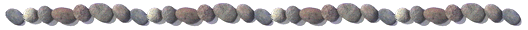Homotopy Analysis MethodThe homotopy analysis method (HAM) is an analytic approximation method for highly nonlinear equations in science, finance and engineering.  It was first proposed by Dr. Shijun LIAO in 1992 in his PhD dissertation, and modified and developed by Dr. Liao with his team and researchers in many other countries.

Motivations

Perturbation techniques are widely applied to gain analytic approximations of nonlinear equations.  However, perturbation methods are essentially based on small physical parameters (called perturbation quantity), but unfortunately many nonlinear problems have no such kind of small physical parameters at all.  In addition, neither perturbation techniques nor the traditional non-perturbation techniques (such as Lyapunov artificial small-parameter method, Adomian decomposition method, delta-expansion method and so on) can provide a way to guarantee the convergence of approximation series.  Therefore, both perturbation techniques and the traditional non-perturbation methods mentioned above are in essence valid only for weakly nonlinear problems.

Advantages of the homotopy analysis method

Based on a generalized concept of the homotopy in topology, the HAM has the following advantages:

The HAM is always valid no matter whether there exist small physical parameters or not;

The HAM provides a convenient way to guarantee the convergence of approximation series;

The HAM provides great freedom to choose the equation type of linear sub-problems and the base functions of solutions.

As a result, the HAM overcomes the restrictions of all other analytic approximation methods mentioned above, and is valid for highly nonlinear problems.

Some new concepts and theorems

Dr. Liao proposed some new concepts in the frame of the HAM, as mentioned below.

1.      Convergence Control

In the frame of the HAM, the so-called convergence-control parameter is introduced for the first time by Dr. Liao in 1997.  This auxiliary parameter has no physical meanings, but provides us a convenient way to guarantee the convergence of approximation series.  In 1999, Dr. Liao further generalized the HAM by introducing more such kind of auxiliary parameters to enlarge the ability of guaranteeing the convergence of approximation series.

2.      Rule of Solution Expression

Based on a generalized concept of homotopy proposed by Dr. Liao in 1997, the HAM transfers a nonlinear problem into an infinite number of linear sub-problems with great freedom to choose the corresponding equation-type and solution expressions.  Unlike perturbation techniques, the starting-point of the HAM is to choose a proper, complete set of base functions to express solutions, according to the physical background or the given boundary/initial conditions or asymptotic properties of considered problems.  The so-called zeroth-order deformation equation (which is a kind of homotopy) should be constructed in such a way that every equation of high-order approximations has a unique solution that obeys the given solution-expression.  This is so important that it becomes a rule in the frame of the HAM, i.e. the rule of solution expression.

The Rule of Solution Expression simplifies the use of the HAM, since it provides a guide to choose the equation type and base functions of linear sub-problems.  Besides, using the Rule of Solution Expression, many unknown physical parameters (such as the period of a nonlinear oscillator) can be easily obtained.  In addition, using the Rule of Solution Expression, some equations of high-order approximations can be solved in a much easier way.

3.      Homotopy Transform

In the frame of the HAM, Dr. Liao deduced a kind of new transform, called the homotopy transform, and further proved that the famous Euler transform is only a special case of the homotopy transform.  This provides us a mathematical cornerstone for the reasonableness of the HAM and for its general validity.